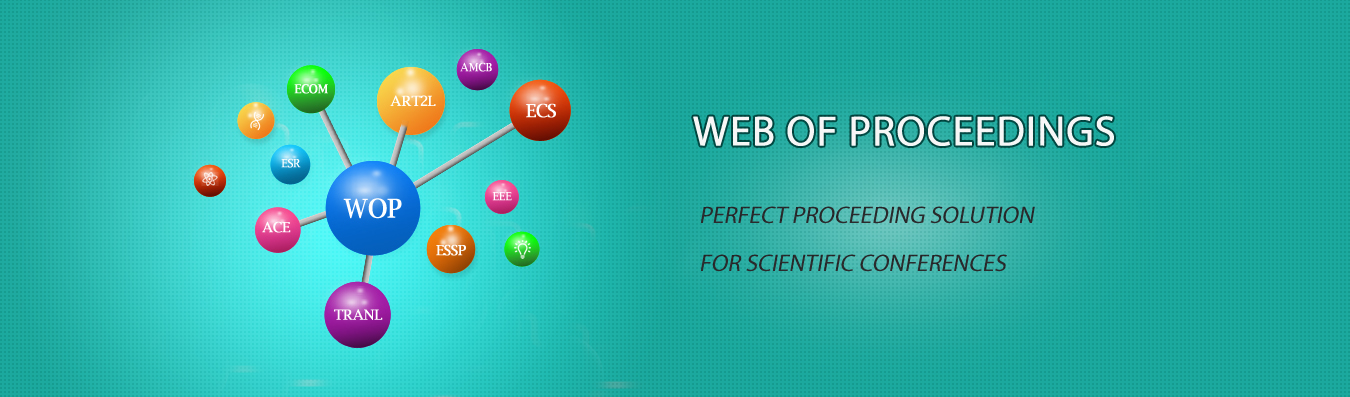The best way to conference proceedings by Francis Academic Press### Application Analysis of Fuzzy Mathematics Based on Comprehensive Evaluation in Mathematical Modeling

DOI: 10.25236/ictmic.2020.043

Wu Wang

Wu Wang

#### Abstract

Fuzzy mathematics is a science to study and deal with fuzzy phenomena, which reveals an uncertainty in division caused by the intermediate transition of differences between objective things. The significance of mathematical modeling lies in the application of mathematical theory to practical problems. As a relatively new theory, fuzzy mathematics has its own huge application background. The significance of mathematical modeling lies in the application of mathematical theory to practical problems. As a new theory, fuzzy mathematics has its huge application background. By introducing the fuzzy mathematical method into the mathematical modeling, we can get some mathematical models which are simpler in calculation and more suitable for the actual situation. Based on the comprehensive evaluation method, this paper discusses the application of fuzzy mathematics in mathematical modeling.

#### Keywords

Fuzzy mathematics, Mathematical modeling, Uncertainty